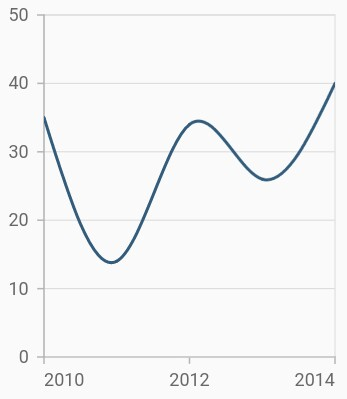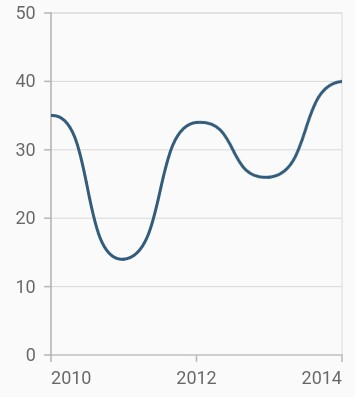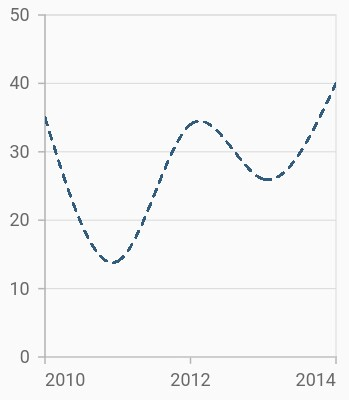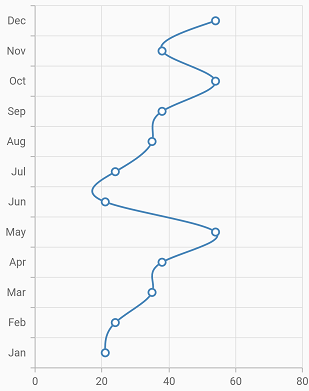# Spline Chart in Flutter Cartesian Charts (SfCartesianChart)

11 Apr 202215 minutes to read

To render a spline chart, create an instance of `SplineSeries`, and add it to the `series` collection property of `SfCartesianChart`. The following properties can be used to customize the appearance of spline segment:

``````@override
Widget build(BuildContext context) {
final List<ChartData> chartData = [
ChartData(2010, 35),
ChartData(2011, 13),
ChartData(2012, 34),
ChartData(2013, 27),
ChartData(2014, 40)
];
return Scaffold(
body: Center(
child: Container(
child: SfCartesianChart(
series: <ChartSeries>[
// Renders spline chart
SplineSeries<ChartData, int>(
dataSource: chartData,
xValueMapper: (ChartData data, _) => data.x,
yValueMapper: (ChartData data, _) => data.y
)
]
)
)
)
);
}
}
class ChartData {
ChartData(this.x, this.y);
final int x;
final double? y;
}``````## Spline rendering types

The `splineType` allows you to change the spline curve in series. The following types can be used in `SplineSeries`:

• natural
• monotonic
• cardinal
• clamped

By default, the value of `splineType` is `natural`.

The following code sample demonstrates how to set the `splineType` value to `cardinal`. When you set the cardinal type, you can specify the desired line tension of the `cardinal` spline using the `cardinalSplineTension` property. The value of this property ranges from 0 to 1.

``````@override
Widget build(BuildContext context) {
final List<ChartData> chartData = [
ChartData(2010, 35),
ChartData(2011, 13),
ChartData(2012, 34),
ChartData(2013, 27),
ChartData(2014, 40)
];
return Scaffold(
body: Center(
child: Container(
child: SfCartesianChart(
series: <ChartSeries>[
SplineSeries<ChartData, int>(
dataSource: chartData,
// Type of spline
splineType: SplineType.cardinal,
cardinalSplineTension: 0.9,
xValueMapper: (ChartData data, _) => data.x,
yValueMapper: (ChartData data, _) => data.y
)
]
)
)
)
);
}
}``````## Dashed spline

The `dashArray` property of the `SplineSeries` is used to render spline series with dashes. Odd value is considered as rendering size and even value is considered as gap.

``````@override
Widget build(BuildContext context) {
final List<ChartData> chartData = [
ChartData(2010, 35),
ChartData(2011, 13),
ChartData(2012, 34),
ChartData(2013, 27),
ChartData(2014, 40)
];
return Scaffold(
body: Center(
child: Container(
child: SfCartesianChart(
series: <ChartSeries>[
SplineSeries<ChartData, int>(
dataSource: chartData,
// Dash values for spline
dashArray: <double>[5, 5],
xValueMapper: (ChartData data, _) => data.x,
yValueMapper: (ChartData data, _) => data.y
)
]
)
)
)
);
}
}``````## Vertical spline chart

The `isTransposed` property of `CartesianSeries` is used to transpose the horizontal and vertical axes, to view the data in a different perspective. Using this feature, you can render vertical Spline chart.

``````@override
Widget build(BuildContext context) {
final List<ChartData> chartData = <ChartData>[
ChartData('Jan', 21),
ChartData('Feb', 24),
ChartData('Mar', 35),
ChartData('Apr', 38),
ChartData('May', 54),
ChartData('Jun', 21),
ChartData('Jul', 24),
ChartData('Aug', 35),
ChartData('Sep', 38),
ChartData('Oct', 54),
ChartData('Nov', 38),
ChartData('Dec', 54)
];
return Scaffold(
body: Center(
child: Container(
child: SfCartesianChart(
isTransposed: true,
primaryXAxis: CategoryAxis(),
series: <ChartSeries>[
SplineSeries<ChartData, String>(
dataSource: chartData,
xValueMapper: (ChartData data, _) => data.x,
yValueMapper: (ChartData data, _) => data.y
)
]
)
)
)
);
}
}
class ChartData {
ChartData(this.x, this.y);
final String x;
final double? y;
}``````